# sfepy.mechanics.matcoefs module¶

Conversion of material parameters and other utilities.

class sfepy.mechanics.matcoefs.ElasticConstants(young=None, poisson=None, bulk=None, lam=None, mu=None, p_wave=None, _regenerate_relations=False)[source]

Conversion formulas for various groups of elastic constants. The elastic constants supported are:

•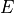: Young’s modulus

•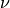: Poisson’s ratio

•: bulk modulus

•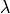: Lamé’s first parameter

•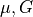: shear modulus, Lamé’s second parameter

•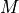: P-wave modulus, longitudinal wave modulus

The elastic constants are referred to by the following keyword arguments: young, poisson, bulk, lam, mu, p_wave.

Exactly two of them must be provided to the __init__() method.

Examples

• basic usage:

>>> from sfepy.mechanics.matcoefs import ElasticConstants
>>> ec = ElasticConstants(lam=1.0, mu=1.5)
>>> ec.young
3.6000000000000001
>>> ec.poisson
0.20000000000000001
>>> ec.bulk
2.0
>>> ec.p_wave
4.0
>>> ec.get(['bulk', 'lam', 'mu', 'young', 'poisson', 'p_wave'])
[2.0, 1.0, 1.5, 3.6000000000000001, 0.20000000000000001, 4.0]

• reinitialize existing instance:

>>> ec.init(p_wave=4.0, bulk=2.0)
>>> ec.get(['bulk', 'lam', 'mu', 'young', 'poisson', 'p_wave'])
[2.0, 1.0, 1.5, 3.6000000000000001, 0.20000000000000001, 4.0]

get(names)[source]

Get the named elastic constants.

init(young=None, poisson=None, bulk=None, lam=None, mu=None, p_wave=None)[source]

Set exactly two of the elastic constants, and compute the remaining. (Re)-initializes the existing instance of ElasticConstants.

class sfepy.mechanics.matcoefs.TransformToPlane(iplane=None)[source]

Transformations of constitutive law coefficients of 3D problems to 2D.

tensor_plane_stress(c3=None, d3=None, b3=None)[source]

Transforms all coefficients of the piezoelectric constitutive law from 3D to plane stress problem in 2D: strain/stress ordering: 11 22 33 12 13 23. If d3 is None, uses only the stiffness tensor c3.

Parameters
c3array

The stiffness tensor.

d3array

The dielectric tensor.

b3array

The piezoelectric coupling tensor.

sfepy.mechanics.matcoefs.bulk_from_lame(lam, mu)[source]

Compute bulk modulus from Lamé parameters.sfepy.mechanics.matcoefs.bulk_from_youngpoisson(young, poisson, plane='strain')[source]

Compute bulk modulus corresponding to Young’s modulus and Poisson’s ratio.

sfepy.mechanics.matcoefs.lame_from_stiffness(stiffness, plane='strain')[source]

Compute Lamé parameters from an isotropic stiffness tensor.

sfepy.mechanics.matcoefs.lame_from_youngpoisson(young, poisson, plane='strain')[source]

Compute Lamé parameters from Young’s modulus and Poisson’s ratio.

The relationship between Lamé parameters and Young’s modulus, Poisson’s ratio (see ,):The plain stress hypothesis:I.S. Sokolnikoff: Mathematical Theory of Elasticity. New York, 1956.

 T.J.R. Hughes: The Finite Element Method, Linear Static and Dynamic Finite Element Analysis. New Jersey, 1987.

sfepy.mechanics.matcoefs.stiffness_from_lame(dim, lam, mu)[source]

Compute stiffness tensor corresponding to Lamé parameters.sfepy.mechanics.matcoefs.stiffness_from_lame_mixed(dim, lam, mu)[source]

Compute stiffness tensor corresponding to Lamé parameters for mixed formulation.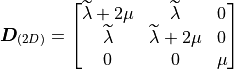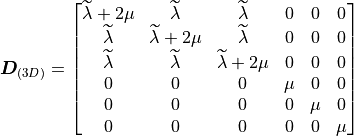wheresfepy.mechanics.matcoefs.stiffness_from_youngpoisson(dim, young, poisson, plane='strain')[source]

Compute stiffness tensor corresponding to Young’s modulus and Poisson’s ratio.

sfepy.mechanics.matcoefs.stiffness_from_youngpoisson_mixed(dim, young, poisson, plane='strain')[source]

Compute stiffness tensor corresponding to Young’s modulus and Poisson’s ratio for mixed formulation.

sfepy.mechanics.matcoefs.youngpoisson_from_stiffness(stiffness, plane='strain')[source]

Compute Young’s modulus and Poisson’s ratio from an isotropic stiffness tensor.